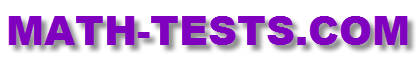#Are you looking for a 6th grade math test that you can take online? The following links provide free access to different tests and quizzes suitable for 6th grade students.

These online tests are designed to work on computers, laptops, iPads, and other tablets. There is no need to download any app for these activities.

Factors
Work with factors and divisibility rules for whole numbers up to 100.

Multiples
Understand the relationship between whole numbers and their multiples.

The Greatest Common Factor (GCF)
Find the greatest common factor of a given set of whole numbers.

Changing Percents to Decimals
Understand the relationship between percents and decimals and how to convert from one to the other by taking this test.

Changing Decimals to Percents
Take this interactive online quiz to practice changing decimals to percents.

Comparing Rational Numbers
This online test gives students the opportunity to compare and order rational numbers in various forms.

The Least Common Multiple (LCM)
Find the least common multiple in this fun online math test activity.

Mixed Numbers and Improper Fractions
Convert mixed numbers to improper fractions in this interactive test of math understanding.

Area of Triangles Test
See how well you can calculate the area of a triangle in this online activity.

Fractions (Review)
Complete this online fractions review in order to check your understanding of basic concepts about fractions.

Changing Fractions to Decimals
Take steps to change a number from fraction form into decimal form, including repeating and non-repeating decimals.

Translations
Translate points, segments, and triangles in the coordinate plane in this online math test.

Return from the 6th Grade Math Test Page to the Math Tests homepage.